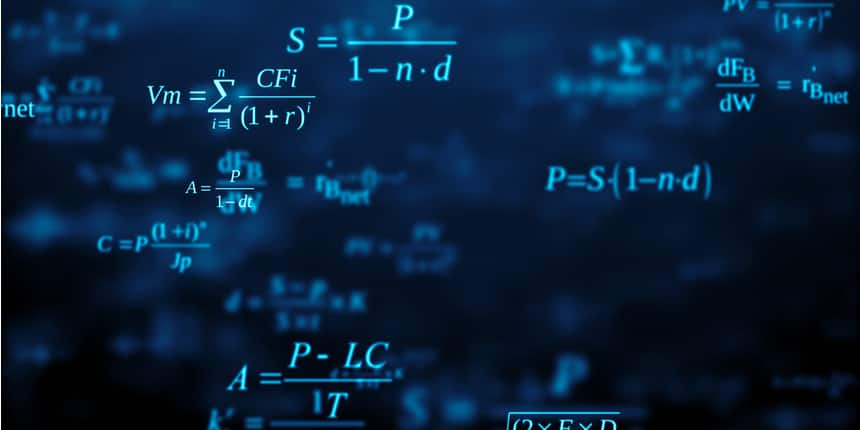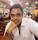Best reference books for CBSE class 10 Mathematics - Class 10th board is the stepping stone of a student’s academic life, being the first biggest exam they attempt. Candidates have to attempt six papers in the CBSE class 10th exam; including Mathematics which is one of the core subjects. Marks in class 10th mathematics goes a long way in deciding the stream a candidate is offered in class 11th. If a student is aiming for the science stream, a good score in Mathematics is imperative. Thus, the preparation for the same must be prioritised. Teachers and exam experts suggest completing the NCERT textbook for Mathematics before attempting any CBSE class 10 Maths reference books.

Mathematics has a total score of 100 marks, of which the exam is for 80 marks and internal assessment is for 20 marks. CBSE 10th question paper can be attempted within 3 hours. The paper has topics such as Number System, Algebra, Geometry, Coordinate Geometry etc. Candidates are advised to divide their preparation according to the exam pattern and weightage of CBSE class 10 Maths exam. The highest weightage is given to Algebra (20 marks) and the second highest weightage is given to Geometry (15 marks).

## CBSE Class 10 Maths Reference Books- Points to consider before buying

Before getting to the best reference books for CBSE class 10 mathematics, it is imperative that students understand what defines a book as a ‘good’ reference book as the matter is very subjective. Here are some qualities of best reference books for CBSE class 10 Maths:

• Easy to understand: It is important for a book to be as easy to understand as possible. The language of a book plays a pivotal role in how much of a topic a student understands. It is also important that the examples and problems given in a book are relatable so as to pique curiosity in students.
• Articulate and concise: Another important thing to consider before wondering about the best reference book for CBSE class 10 mathematics is that it should be articulate and concise. There are many axioms, formulas and concepts which are introduced to students in class 10th. To ensure that students understand them completely, the books must be concise and articulate.
• Engaging and informative diagrams: A good book must have plenty of informative illustrations like graphs, diagrams and tables as they are more fluent for a student to understand. Students can look at graphs and diagrams as they help them to understand a problem or a concept better.

## Best Reference Books for CBSE Class 10 Maths

After checking the syllabus, students can look at the list of CBSE class 10 Mathematics - best reference books. The list is divided into segments like books prescribed by CBSE, books used by previous year toppers, books prescribed by leading coaching institutes and such. Read the list down for best reference books for CBSE class 10 Mathematics:

## CBSE Class 10th Maths Reference Books

Central Board of Secondary Education(CBSE) conducts the CBSE boards for class 10th and recommends the students to be taught and tested in questions based on the following books. NCERT books are helpful in building the basic concepts of students and it is important to solve the complete NCERT textbook along with the examples and then progress towards reference books. After solving Mathematics - Textbook for class X by NCERT, the students should move on to solve Mathematics Exemplar Problems for class 10 by NCERT. The questions in Mathematics Exemplar Problems are more advanced and conceptual. Here’s the complete list of books prescribed by CBSE Board:

CBSE Prescribed books for 10th Board

 S.No. Book Author 1. Mathematics - Textbook for class X NCERT Publication 2. Mathematics exemplar problems for class X NCERT publication 3. Guidelines for Mathematics Laboratory in Schools, class X CBSE Publication 4. Laboratory Manual - Mathematics, the secondary stage NCERT Publication

## Best Reference Books for CBSE Class 10 Maths (Recommended by Toppers)

The following books have been tried, tested and trusted by many exam experts and toppers who prepared using these reference books for CBSE class 10 Mathematics. Check out the complete table given below:

Toppers’ prescribed best CBSE class 10 Mathematics reference books

 S.No. Book Author 1. Mathematics for Class 10 R. D. Sharma 2. Secondary School Mathematics for Class 10 R. S. Aggarwal 3. All in one mathematics Arihant

### CBSE Class 10 Mathematics Syllabus

Before delving into the best reference books for CBSE class 10 Mathematics, aspirants must know the complete CBSE syllabus for class 10. This will help them in selecting the best mathematics reference books for CBSE class 10. Check the table below to read the complete syllabus:

 S.No Unit Marks 1 Number System 6 2 Algebra 20 3 Coordinate Geometry 6 4 Geometry 15 5 Trigonometry 12 6 Mensuration 10 7 Statistics & Probability 11 Total Marks 80

### How to Prepare for CBSE Board Class 10 Maths?

Mathematics requires practice and your attitude towards it can dramatically change depending on your practice. Coaching institutes analyse students who attend their centres and compile preparation tricks, which are helpful for students. Thus, there are a few preparation tips and strategies they suggest all the students. Here are a few preparation tips for CBSE class 10 Maths Board exam:

• Go through the CBSE Board 10th exam pattern - When we talk about best reference books for CBSE class 10 Mathematics, exam pattern of Mathematics exam comes to the mind. Students should be aware of the exam pattern as it will give them an insight into the typology of questions and the distribution of marks. Exam pattern also helps you to understand what type of questions and topics will be asked from a particular unit/chapter. The exam pattern for class 10th Maths board is given below:
• Mathematics exams are divided into two papers, internals, for 20 marks and the Board exam paper for 80 marks.
• Internals will include a periodic test of 10 marks, notebook submission of 5 marks and subject enrichment activities of 5 marks.
• Mathematics exam for 80 marks will include Very Short Answer Type Questions (VSA), Short Answer Type Questions I & II (SA) and Long Answer Type Questions (LA). This paper will evaluate the knowledge, understanding, application and analysis of students’ Maths knowledge.
• To clear the paper, students will have to score at least 30% in each paper (internals & board exam).
• Solve the complete NCERT textbook - NCERT textbook is the primary book for class X Boards and it is very important to solve this book as many students have reported that questions are conceptually based on NCERT textbook exercises and textbook. Thus, before moving to the best reference books for CBSE class 10 Mathematics, remember to solve questions from NCERT.
• Work on your basics and concept - Focus and clear your fundamental concepts before moving on towards the more advanced topics. This will give you a concrete ground on which you can build your knowledge on further studies and help you in understanding the concepts better during your 11th, 12th and even competition level Mathematics.
• Make flashcards for theorems, derivations and formulas - Mathematics is full of theorems, derivations and formulas. From the derivation of Pythagoras Theorem to formulas of Trigonometry, everything is important to learn to make the fundamentals strong for further studies. However, many students struggle with remembering all these theorems and formulas and tend to forget them. Instead of going through the trouble of opening the textbook or notebook and going through it again and again, make flashcards and notes so that all of these are more accessible.
• Focus on learning how to solve a problem along with accuracy - Where competition exams focus on accuracy, CBSE uses the step-wise method to check copies. This means for questions ranging from 2-4 marks, the examiner will focus more on the method you use to solve a problem than if your answer is correct or not, thus you should focus on how to solve the problem along with accuracy. Also, remember to do your rough work at the side of the paper by making a margin so that it looks more authentic.

Entrance Exams

 Medical Entrance Exams Engineering Entrance Exams Scholarship Exam NEET-UG JEE Main KVPY Scholarship Exam JIPMER MBBS JEE Advanced NTSE Scholarship Exam

## Questions related to CBSE Class 10th

Showing 2 out of 2 Questions
15 Views

## How can I cancel my Improvement form of class 10th?Tamajit Student Expert 23rd Oct, 2019As per CBSE guidelines, there is no mention of a methodology for cancelling your improvement examination or nullify your candidature for the improvement examination. However, what you can do is you can skip the improvement examination only if you do not want to appear for the examination.

CBSE generates two separate marksheets for improvement candidates: one during the normal attempt of the examination and the other after the improvement examination which carries the marks of the improvement examination, thus you can show the mark sheet of your first or normal attempt and that way there is no evidence to prove the fact that you applied for the improvement examination. But do keep in mind that you must have an explanation for the academic gap that you are going to have between class X and class XII as that might be asked in your future places of academic enrollment.

BEST WISHES!

9 Views

## can i opt for biocomputer after selecting basic maths in class 10 th

Sunanda Dhar Student Expert 24th Sep, 2019yes you can opt for biocomputer after having maths at class 10.At class 10 leve basics are taught so can easily copeup with that.